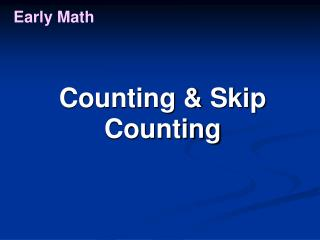DownloadDownload PresentationEarly Math

# Early Math

Télécharger la présentation## Early Math

- - - - - - - - - - - - - - - - - - - - - - - - - - - E N D - - - - - - - - - - - - - - - - - - - - - - - - - - -
##### Presentation Transcript

1. Early Math Counting & Skip Counting

2. Early Math Counting & Skip Counting “0” – see the number

3. Early Math Counting & Skip Counting Now count and move one

4. Early Math Counting & Skip Counting

5. Early Math Counting & Skip Counting “1” – see the number

6. Early Math Counting & Skip Counting Now count and move one more

7. Early Math Counting & Skip Counting “2” – see the number

8. Early Math Counting & Skip Counting Now count and move one more

9. Early Math Counting & Skip Counting “3” – see the number

10. Early Math Counting & Skip Counting Now count and move one more

11. Early Math Counting & Skip Counting “4” – see the number

12. Early Math Counting & Skip Counting Now count and move one more

13. Early Math Counting & Skip Counting “5” – see the number

14. Early Math Counting & Skip Counting Now count and move one more

15. Early Math Counting & Skip Counting “6” – see the number

16. Early Math Counting & Skip Counting Now count and move one more

17. Early Math Counting & Skip Counting “7” – see the number

18. Early Math Counting & Skip Counting Now count and move one more

19. Early Math Counting & Skip Counting “8” – see the number

20. Early Math Counting & Skip Counting Now count and move one more

21. Early Math Counting & Skip Counting “9” – see the number

22. Early Math Counting & Skip Counting Now count and move one more

23. Early Math Counting & Skip Counting “10” – see the number

24. Early Math Counting & Skip Counting - Count by 2’s Move two at a time on MathLine

25. Early Math Counting & Skip Counting - Count by 2’s Now count and move two

26. Early Math Counting & Skip Counting - Count by 2’s “2” – see the number

27. Early Math Counting & Skip Counting - Count by 2’s Now count and move two more

28. Early Math Counting & Skip Counting - Count by 2’s “4” – see the number

29. Early Math Counting & Skip Counting - Count by 2’s Now count and move two more

30. Early Math Counting & Skip Counting - Count by 2’s “6” – see the number

31. Early Math Counting & Skip Counting - Count by 2’s Now count and move two more

32. Early Math Counting & Skip Counting - Count by 2’s “8” – see the number

33. Early Math Counting & Skip Counting - Count by 2’s Now count and move two more

34. Early Math Counting & Skip Counting - Count by 2’s “10” – see the number

35. Early Math Counting & Skip Counting - Count by 3’s Move three at a time on MathLine

36. Early Math Counting & Skip Counting - Count by 3’s Now count and move three

37. Early Math Counting & Skip Counting - Count by 3’s “3” – see the number

38. Early Math Counting & Skip Counting - Count by 3’s Now count and move three

39. Early Math Counting & Skip Counting - Count by 3’s “6” – see the number

40. Early Math Counting & Skip Counting - Count by 3’s Now count and move three

41. Early Math Counting & Skip Counting - Count by 3’s “9” – see the number

42. Early Math Counting & Skip Counting - Count by 3’s Now count and move three

43. Early Math Counting & Skip Counting - Count by 3’s “12” – see the number

44. Early Math Counting & Skip Counting - Count by 3’s Now count and move three

45. Early Math Counting & Skip Counting - Count by 3’s “15” – see the number

46. Early Math Counting & Skip Counting - Count by 3’s Now count and move three

47. Early Math Counting & Skip Counting - Count by 3’s “18” – see the number

48. Early Math Remember MathLine can be used in all counting, skip counting and counting backwards activities. These could be done as a class, small group or by an individual.